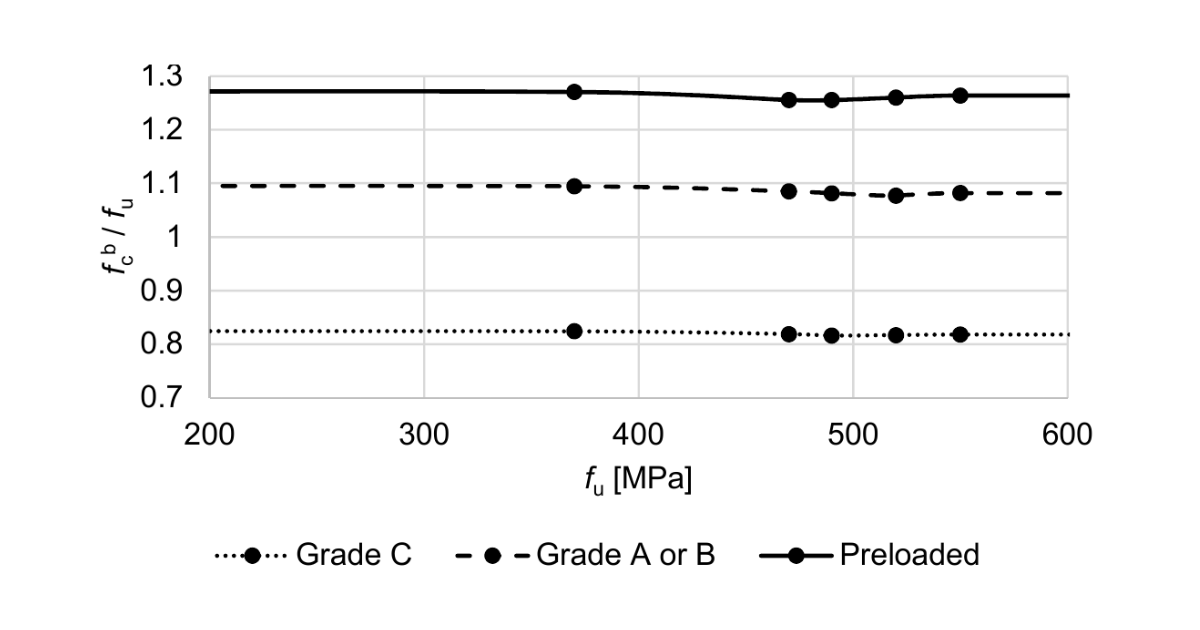### Choose language# Code-check of bolts according to Hong Kong Code

$$Bolts in tensionBolt resistance in tension is checked according to Cl. 9.3.7.1 as:$P_t = A_s \cdot p_t$where: $$A_s$$ – tensile stress area $$p_t$$ – tension strength obtained from Table 9.8 Prying forces are taken into account by finite element analysis.Bolts in shearShear capacity of bolts is taken according to Cl. 9.3.6.1.1 as:$P_s = p_s \cdot A_s$where: $$p_s$$ – design shear strength obtained from Table 9.5 $$A_s$$ – effective shear area; $$A_s = A_t$$ if threads are intercepted by shear plane, $$A_s$$ is taken as the cross sectional area of the shank otherwise $$A_t$$ – tensile area According to Cl. 9.3.6.1.6, when a bolt passes through packing with thickness $$t_{pa}$$ greater than one-third of the nominal diameter $$d$$, its shear capacity $$P_s$$ should be reduced by multiplying a reduction factor $$\beta_p$$ obtained from:$\beta_p = \frac{9d}{8d+3t_{pa}} \le 1$Bolts in combined tension and shearCombined tension and shear is checked according to Cl. 9.3.8.1 as:$\frac{F_s}{P_s} + \frac{F_{tot}}{P_t} \le 1.4$where: $$F_s$$ – shear force in a bolt $$P_s$$ – shear resistance of a bolt $$F_{tot}$$ – total applied tension in the bolt including the prying force $$P_t$$ – tensile resistance of a bolt Bolts in bearingBearing capacity of bolts is taken according to Cl. 9.3.6.1.2 as:$P_{bb} = d \cdot t_p \cdot p_{bb}$where: $$d$$ – nominal diameter of the bolt $$t_p$$ – thickness of the connected plate $$p_{bb}$$ – bearing strength of the bolt obtained from Table 9.6 Each plate is checked separately and the worst result is shown.Bearing capacity of connected parts is taken according to Cl. 9.3.6.1.3 as the minimum of the following:$P_{bs} = k_{bs} \cdot d \cdot t_p \cdot p_{bs}$$P_{bs} = 0.5 \cdot k_{bs} \cdot e \cdot t_p \cdot p_{bs}$$P_{bs} = 1.5 \cdot l_c \cdot t_p \cdot U_s \le 2.0 \cdot d \cdot t_p \cdot U_b$where: $$k_{bs}$$ – hole coefficient taken as for standard holes $$k_{bs} = 1.0$$ for oversized and short slotted holes $$k_{bs} = 0.7$$ for long slotted holes $$k_{bs} = 0.5$$ $$d$$ – nominal bolt diameter $$t_p$$ – connected plate thickness $$p_{bs}$$ – bearing strength of connected parts for steel of grade S275, $$p_{bs} = 460$$ MPa for steel of grade S355, $$p_{bs} = 550$$ MPa for steel of grade S460, $$p_{bs} = 670$$ MPa for steel of other grades, $$p_{bs} = 0.67 (U_s+Y_s)$$ $$e$$ – edge distance in the direction of the shear force measured from the centreline of the bolt $$l_c$$ – net distance between the bearing edge of the holes and the near edge of adjacent hole in the same direction of load transfer $$U_s$$ – minimum tensile strength of connected plate $$Y_s$$ – characteristic yield strength of connected plate $$U_b$$ – specified minimum tensile strength of bolt$$